Courses

# Break Even Analysis - Marginal Costing, Cost Management B Com Notes | EduRev

## B Com : Break Even Analysis - Marginal Costing, Cost Management B Com Notes | EduRev

The document Break Even Analysis - Marginal Costing, Cost Management B Com Notes | EduRev is a part of the B Com Course Cost Management.
All you need of B Com at this link: B Com

#### 1. Meaning of Break-Even Analysis:

The study of cost-volume profit analysis is often referred to as ‘break-even analysis’ and the two terms are used interchangeably by many. This is so, because break-even analysis is the most widely known form of cost-volume-profit analysis. The term “break-even analysis” is used in two senses—narrow sense and broad sense.

In its broad sense, break-even analysis refers to the study of relationship between costs, volume and” profit at different levels of sales or production. In its narrow sense, it refers to a technique of determining that level of operations where total revenues equal total expenses, i.e., the point of no profit, no loss.

#### 2. Assumptions of Break-Even Analysis:

The break-even analysis is based upon the following assumptions:

(i) All elements of cost, i.e., production, administration and selling and distribution can be segregated into fixed and variable components.

(ii) Variable cost remains constant per unit of output irrespective of the level of output and thus fluctuates directly in proportion to changes in the volume of output.

(iii) Fixed cost remains constant at all volumes of output.

(iv) Selling price per unit remains unchanged or constant at all levels of output.

(v) Volume of production is the only factor that influences cost.

(vi) There will be no change in the general price-level.

(vii) There is only one product or in case of multi-products, the sales mix remains unchanged.

(viii) There is synchronisation between production and sales.

#### 3. Break Even Point:

The break-even point may be defined as that point of sales volume at which total revenue is equal to total cost. It is a point of no profit, no loss. A business is said to break-even when its total sales are equal to its total costs. The break-even point refers to that level of output which evenly breaks the costs and revenues and hence the name.

At this point, contribution, i.e., sales minus marginal cost, equals the fixed costs and hence this point is often called as ‘Critical Point’ or ‘Equilibrium Point’ or ‘Balancing Point’ or no profit, no loss. If production/sales is increased beyond this level, there shall be profit to the organisation and if it is decrease from this level, there shall be loss to the organisation.

Break-even point can be stated in the form of an equation:

Sales revenue at break-even point = Fixed Costs + Variable Costs.

Computation of the Break-Even Point:

The break-even point can be computed by the following methods:

(i) The Algebraic Formula Method

(ii) Graphic or Chart Method.

Algebraic Formula Method for Computing the Break-Even Point:

The break-even point can be computed in terms of:

(a) Units of sales volume.

(b) Budget total or in terms of money value.

(c) As a percentage of estimated capacity.

(a) Break-Even Point in Units:

As the break-even point is the point of no profit no loss, it is that level of output at which the total contribution equals the total fixed costs, It can be calculated with the help of following formula: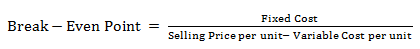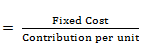(b) Break-even Point in terms of budget-total or money value: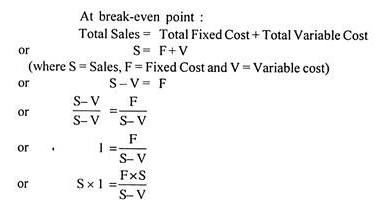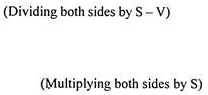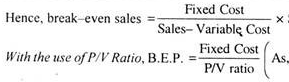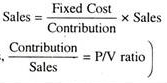(c) Break-even Point as a percentage of estimated capacity:

Break-even point can also be computed as a percentage of the estimated sales or capacity by dividing the break-even sales by the capacity sales. For example, if a firm has an estimated capacity of 1,00,000 units of products and its break-even point is reached at 50,000 units, then the break-even point is at 50% of capacity (1,00,000/50,000).

If information as to total contribution at full capacity is available, the break-even point as a percentage of estimated capacity can be found as under:

B.E.P (as % age of capacity) = Fixed Cost/Total Contribution

Illustration 1:

From the following information, calculate the break-even point in units and in sales value:

Output = 3,000 units

Selling price per unit = Rs. 30

Variable cost per unit =Rs. 20

Total fixed cost = Rs. 20,000

Solution: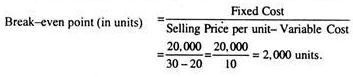#### 4. Types of Break-Even Point:

(i) Cash Break-Even Point:

In the present competitive world of business, it may be difficult for new industrial units to achieve the break-even point in the initial years. Thus, the concept of cash break-even point has emerged. The cash break­even point may be defined as that point of sales volume at which total revenue is equal to total cash cost.

At this point, cash contribution (which is calculated after making adjustment for variable portion of depreciation, etc.) equals the cash fixed cost, i.e., fixed cost excluding depreciation and deferred expenses. This point enables the management to determine the level of activity below which the liquidity position of the firm would be adversely affected.

Thus, cash break-even point may be calculated as below:

Cash Break- Even Point (in Units) = Cash Fixed Cost/Cash Contribution per unit

Illustration 2:

From the following information, calculate the cash Break-Even Point: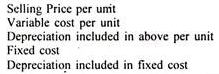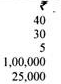Solution:

(ii) Composite Break-Even Point:

So far we have dealt with break-even point of firms producing single product. We can also calculate the composite break-even point for a firm producing several products, as below:

Composite Break-Even Point (in Sales value) = Total Fixed Cost/Composite P/V Ratio

and, Composite P/V Ratio = Total Contribution/Total Sales × 100

Illustration 3:

From the following information of a company producing three products, you are required to compute:

(a) Composite P/V Ratio, and

(b) Composite Break-Even Point.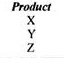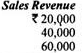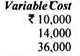Fixed costs: Rs. 50,000.

Solution: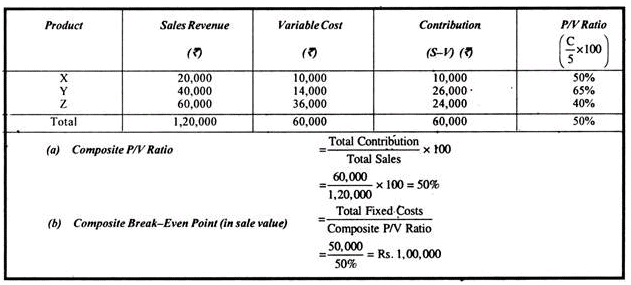#### 5. Graphic Method of Break-Even Analysis:

The break-even point can also be computed graphically. A break-even chart is a graphical representation of marginal costing. The break-even chart ‘Portrays a pictorial view of the relationships between costs, volume and profits.’

It shows the break-even point and also indicates the estimated profit or loss at various levels of output. The break-even point as “indicated in the chart is the point at which the total cost line and the total sales line intersect.

There are three methods of drawing a break-even chart. These methods of drawing break-even chart have been explained with the help to the following illustration.

Illustration 4:

Plot the following data on a graph (break-even chart) and determine:

(a) Break-even point

(b) Profit if the output is 25,000 units.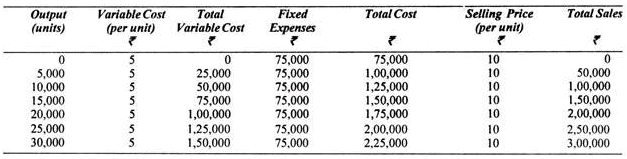Solution:

First Method:

Under this method following steps are taken to draw the break-even chart:

i. Volume of production/output or sales is plotted on horizontal axis, i.e., X-axis. The volume of sales or production may be expressed in terms of rupees, units or as a percentage of capacity.

ii. Costs and sales revenue are represented on vertical axis, i.e., Y-axis.

iii. Fixed cost line is drawn parallel to X-axis. The line indicates that fixed expenses remain constant at all levels of activity.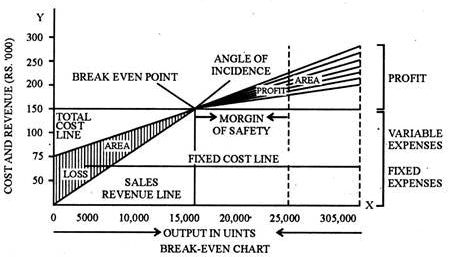iv. The variable costs for different levels of activity are plotted over-the fixed cost line. The variable cost line is joined to fixed cost line at zero level of activity. As the variable cost line is drawn above the fixed cost line, it represents the total cost at various levels of output/sales.

v. Sales values at various levels of output are plotted and a line is drawn joining these plotted points. This line is called the sales (revenue) line.

vi. The point of intersection of total cost line and sales (revenue) line is called the break-even point.

vii. The number of units to be produced at break-even point can be determined by drawing a perpendicular to the X-axis from the point of intersection of cost and sales line.

viii. The sales revenue at break-even point can be determined by drawing a perpendicular to the X- axis from the point of inter-section of cost and sales line.

ix. The area below the break-even point represents the loss area as the total sales and less than the total cost and the area above the break-even point indicates the area of profit as the sales revenue exceeds the total cost.

Second Method:

The break-even chart can also be drawn by another method which is a variation of the first method. Under this method, the variable cost line is drawn first and then fixed cost line is drawn over and parallel to le variable cost line. The fixed cost line, so drawn, represents the total cost (Variable + Fixed) at various levels of output because it is drawn above the variable cost line.

This method is useful to the management for decision taking because it reveals additional information:

(a) The variable costs are shown directly for various levels of output/sales.

(b) Marginal contribution at various levels of sales is indicated clearly by the difference between sales line and variable cost line.

(c) It indicates the recovery of fixed costs at various levels of production.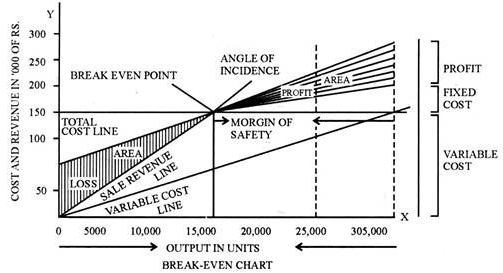A small variation of this method is that of showing the various elements of fixed and variable costs, for example, major cost elements such as direct material cost, labour cost, variable factory overhead cost, variable selling overhead and fixed costs.

Third Method—Contribution Break-even Chart:

This is a modified form of a simple break-even chart as shown in the first-two methods above. Under this method total cost line is not drawn, rather another line called contribution line is drawn from the origin and this line goes up with the increase in the level of output. The fixed cost line is drawn parallel to the x-axis as in the first method.

The sales line is also drawn as usual. In this method, the question of intersection of sales line with the total cost line does not arise because there is no cost line. The break-even point is that point where the contribution line crosses the fixed cost line. At this point, total contribution is equal to the total fixed cost and hence there is no profit or loss.

As the contribution increases more than the fixed cost, profit shall arise to the organisation and as contribution decreases from the fixed cost, there shall be loss to the organisation. The contribution break-even chart shows clearly contribution at different levels of activity and indicates that all levels below the break-even point are unable to cover the fixed costs.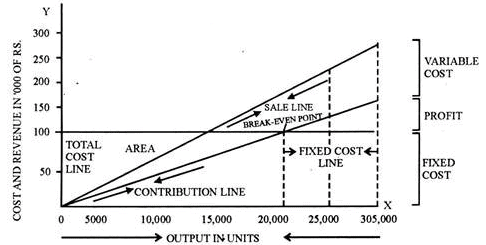In the above example, at level of output/sales of 25,000 units, there is a profit of Rs. 50,000 as indicated by the break-even charts under the three methods.

#### 6. Assumptions Underlying Break-Even Charts:

There are a number of assumptions which are made while drawing a break-even chart, such as:

(i) All costs can be separated into fixed and variable costs.

(ii) Fixed costs remain constant at all levels of activity.

(iii) Variable cost fluctuates directly in proportion to changes in the volume of output.

(iv) Selling prices per unit remain constant at all levels of activity.

(v) There is no opening or closing stock.

(vi) There will be no change in operating efficiency.

(vii) Product mix remains unchanged or there is only one product.

(viii) The volume of output or production is the only factor which influences the cost.

Computation of break-even point or presentation of cost, volume and profit relationship by way of break-even charts has the following advantages:

i. Information provided by the break-even chart is in a simple form and is clearly understandable even to a layman. The whole idea of the problem is presented at a glance.

ii. The break-even chart is very useful to the management for taking managerial decisions because the chart studies the relationship of cost, volume and profit at various levels of output. The effects of changes in fixed costs and variables costs at various levels of output and that of changes in the selling price on the profits can be depicted very clearly by way of break-even charts.

iii. The break-even charts help in knowing and analysing the profitability of different products under various circumstances.

iv. A break-even chart is very useful for forecasting (the costs and profits), planning and growth.

v. The break-even chart is a managerial tool for control of costs as it shows the relative importance of fixed cost in the total cost of a product.

vi. Besides determining the break-even point, profits at various levels of output can also be determined with the help of break-even charts.

vii. The break-even charts can also be used to study the comparative plant efficiencies of business.

#### 8. Limitations of Break-Even Charts:

Despite many advantages, a break-even chart suffers from the following limitations:

i. A break-even chart is based upon a number of assumptions, discussed above, which may not hold good under all circumstances. For example, fixed costs do not remain constant after a certain level of activity; variable costs do not always vary in direct proportion to changes in the volume of output because of the laws of diminishing and increasing returns; selling prices do not remain the same forever and for all levels of output due to competition and changes in the general price level; etc.

ii. A break-even chart provides only a limited information. We have to draw a number of charts to study the effects of changes in the fixed costs, variable costs and selling prices on the profitability. In such cases, it becomes rather more complicated and difficult to understand.

iii. Break-even charts present only cost-volume profit relationships but ignore other important considerations such as the amount of capital investment, marketing problems and government policies, etc.

iv. A break-even chart does not suggest any action or remedies to the management as a tool of management decisions.

v. More often, a break-even chart presents only a static view of the problem under consideration.

#### 9. Margin of Safety:

The excess of actual or budgeted sales over the break-even sales is known as the margin of safety. It is the difference between actual sales minus the sales at break-even point. It represents the amount by which sales revenue can fall before a loss is incurred.

As at break-even point there is no profit no loss, sales beyond the break-even point represent margin of safety because any sales above the break-even point will give some profit.

Thus,

Margin of Safety = Total Sales – Sales at Break-Even Point.

Say, actual present sales are Rs. 5,00,000 and the break-even sales are Rs. 4,00,000, then margin of safety is equal to Rs. 1,00,000, i.e., 5,00,000 – 4,00,000.

Margin of Safety can also be expressed in percentage. For example, if a company can break-even at 60 per cent of the expected sales; then it has a margin of safety of (100-60) 40 per cent. In the previous example, margin of safety in percentage can be calculated as 1,00,000/5,00,000 × 100 = 20%

Margin of safety calculated in percentage is also known as Margin of Safety Ratio and can be expressed as:

M.S. Ratio = M.S./Sales × 100

= Actual Sales – Sales at B. E. P./Sales × 100

Margin of safety can also be calculated with the help of the following formula:

Margin of Safety (M/S) = Profit/P/V Ratio

This is so because margin of safety is the volume of sales beyond break-even point and all sales above the break-even point give some profit, which can be calculated as:

Profit = Margin of Safety × P/V ratio

or M.S.= Profit/P/V Ratio

The size of the margin of safety is an important indicator of the strength of a business. The large margin of safety indicates that the business is sound and even if there is a substantial fall in sales, there will still be some profit. On the other hand, small margin of safety indicates that position of the business is comparatively weak and even a small decline in the sales would adversely affect the profit of the business and may result into losses.

The margin of safety can be improved by taking the following steps:

i. By increasing the level of production

ii. By increasing the selling price

iii. By reducing the fixed cost

iv. By reducing the variable cost

v. By substituting unprofitable products with profitable products

vi. By increasing contribution by changing the sales mix or by dropping unprofitable products.

10. Angle of Incidence:

The angle of incidence is the angle between the sales line and the total cost line formed at the break-even point where the sales line and the total cost line intersect each other. The angle of incidence indicates the profit earning capacity of a business. A large angle of incidence indicates a high rate of profit and, on the other hand, a small angle of incidence indicates a low rate of profit.

Usually, the angle of incidence and margin of safety are considered together to indicate the soundness of a business. A large angle ofincidence with a high margin of safety indicates the most favourable position of a business.

#### 11. Profit-Volume Graph:

Profit-volume graph is a pictorial representation of the profit-volume relationship. This graph shows profit and loss at different volumes of sales. It is said to be a simplified form of break-even chart as it clearly represents the relationship of profit to volume of sales.

A profit-volume graph also called the P/V graph or profit graph can be constructed from any data relating to a business from which a break-even chart can be drawn.

The profit-volume graph may be preferred to a break-even chart because profits or losses can be directly read at different levels of activity. But the basic limitation of a P/V graph is that is does not show how costs vary with the change in the level of activity. For this reason, break-even chart and profit-volume graph should both be drawn together to derive the maximum advantage of both.

The construction of a profit-volume graph involves the following steps:

i. Sales line (in volume or value) is drawn on horizontal or x-axis.

ii. Profits and losses are given on vertical or y-axis.

iii. The area above the horizontal of x-axis is called the profit area and the area below the horizontal axis is the loss area.

iv. Profits and losses at different levels of activity are plotted against corresponding sales and then these points are joined and extended. This line is called profit line. In case of more than one product, a separate profit line for each product should be drawn.

v. The point where profit line intersects with the sales line is the break-even point.

Illustration 5:

Prepare a P/V graph from the following data: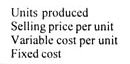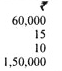Show the expected sales on the graph when the profit to be earned is Rs. 87,500.

Solution: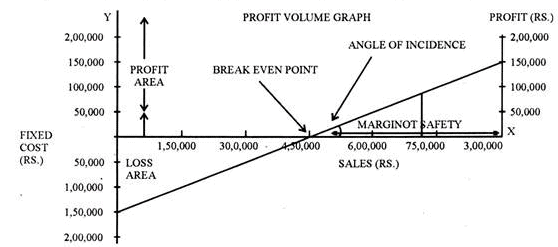Arithmetical Verification:

Sale (in units) = Fixed Expenses + Profit/Contribution per unit

= 1,50,000 + 87,500/5 = 2,37,500/5 = 47,500 units

... Sales = = 47,500 units @ Rs. 15 = Rs. 7,12,500

Illustration 6:

The following figures relate to one year’s working at 100 per cent capacity level in a manufacturing business:

Direct Wages – Rs. 1,50,000

Direct Materials – Rs. 4,10,000

Sales – Rs. 10,00,000

Represent the above figures on a break-even chart and determine from the chart the break-even point.

Solution: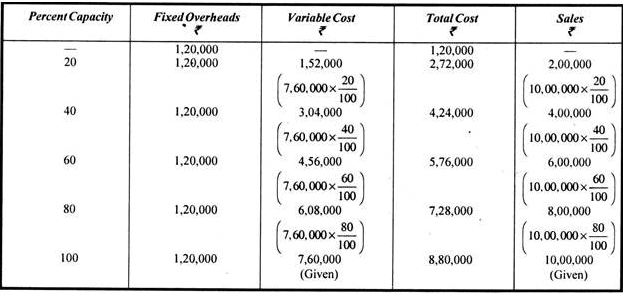Arithmetical Verification: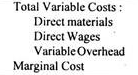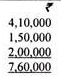Contribution = Sales – Marginal Cost

= Rs. 10,00,000 – Rs. 7,60,000

= Rs. 2,40,000

P/V Ratio = Contribution/Sales

2,40,000/10,00,000 = 24/100 or 24%.

Break-Even Point = Fixed Expenses/P/V Ratio

= 1,20,000/24/100 = 1,20,000 × 100/24 = Rs. 5,00,000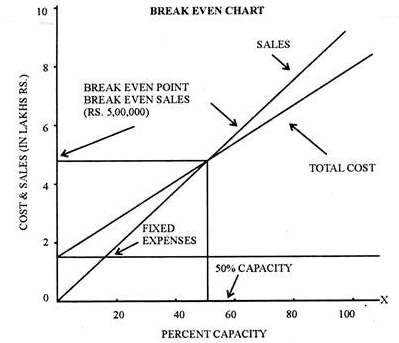At Rs. 10,00,000 sales, 100% capacity is reached

... At Rs. 5,00,000 sales, 50% capacity is reached

Hence, break-even point is reached at a 50% capacity utilisation.

Illustration 7:

You are given the following data for the coming year of a factory: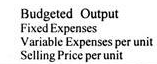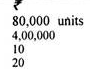Draw a break-even chart showing the break-even point. If the selling price is reduced to Rs. 18 per unit, what will be the new break-even point?

Solution: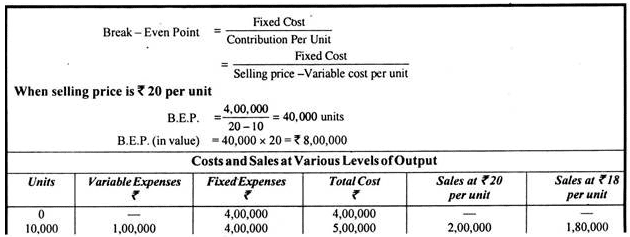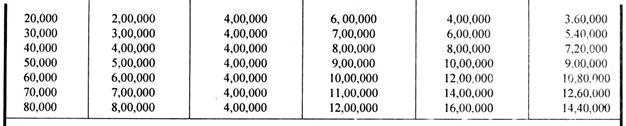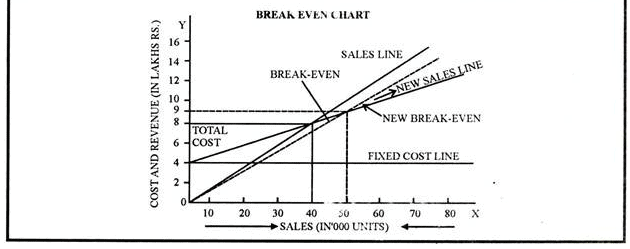Illustration 8:

AB Ltd. and XY Ltd. anticipate sales turnover amounting to Rs. 25,00,000 10% of which is expected to be profit if each achieves 100% of normal capacity. The variable costs are Rs. 13,50,000 for AB Ltd. and Rs. 20,00,000 for XY Ltd.

Present the necessary details graphically on a single break-even chart, and determine therefrom the capacity at each of the break-even points:

Solution: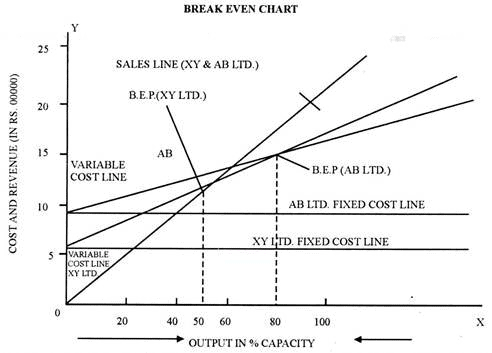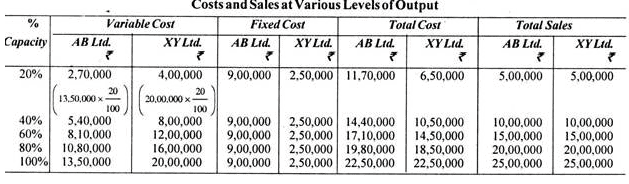#### 12. Curvilinear Break-Even Analysis (Two Break-Even Points):

The marginal costing approach is based upon the basic assumption that selling price and variable cost per unit will remain constant at all levels of activity or in other words the cost-volume-profit relationship is linear. However, in actual practice, the selling prices do not remain the same forever and for all levels of output due to competition and changes in the general price level etc.

Further, it may not be possible to increase the sales volume without offering concessions in price to the customers. In the same manner, variable cost per unit may also increase with the increase in level of production due to operating inefficiencies and the law of diminishing returns.

Thus, profit can be increased only upto a certain point and then it will decrease until it is converted into a loss. The break-even chart will then become curvilinear instead of linear. It might show more than one break-even point, one at a lower level of output and another at a higher level of output.

In such a case, increasing output/sales volume beyond the first break-even point will increase profit but increase in volume beyond the second break-even point will result in loss. The optimum level of output shall be reached at the point where difference between the total revenue and the total cost is the highest.

Offer running on EduRev: Apply code STAYHOME200 to get INR 200 off on our premium plan EduRev Infinity!

## Cost Management

52 videos|51 docs

,

,

,

,

,

,

,

,

,

,

,

,

,

,

,

,

,

,

,

,

,

,

,

,

;